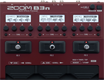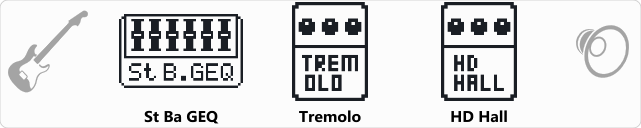# Factory : TxTremolo

Discussion in 'Zoom B3n' started by Tonelib, May 20, 2018.

1. Factory : TxTremoloDevice: Zoom B3n
Firmware: 2.00

Name on device: TxTremolo
Optimized for: Phones/Speaker

Effects chain:Combines a tremolo tone with deep reverb for a back woods sound.

Effect: "St Ba GEQ" (Filter), active - "yes"
"50Hz" = 0.0
"120Hz" = 0.0
"400Hz" = 0.0
"500Hz" = 0.0
"800Hz" = 0.0
"4.5kHz" = 0.0
"10kHz" = 0.0
"Volume" = 80
"50Hz" = 0.0
"120Hz" = 0.0
"400Hz" = 0.0
"500Hz" = 0.0
"800Hz" = 0.0
"4.5kHz" = 0.0
"10kHz" = 0.0
"Volume" = 80
"Parameter set" = A

Effect: "Tremolo" (Modulation), active - "yes"
"Wave" = SQR
"Depth" = 68
"Rate" = 32
"Volume" = 55

Effect: "HD Hall" (Reverb), active - "yes"
"PreD" = 81
"Decay" = 53
"Mix" = 57
"Tail" = Off

Patch Volume: 115